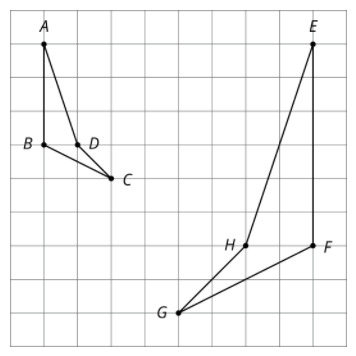PSABM5VW - Practice---Acc7.5 Lesson 6 Representations of Linear Relationships (5.MD.C.3, 8.EE.B, 8.EE.B.6)

Create a graph that shows three linear relationships with different y-intercepts using the following slopes, and write an equation for each line.

Slopes:

•••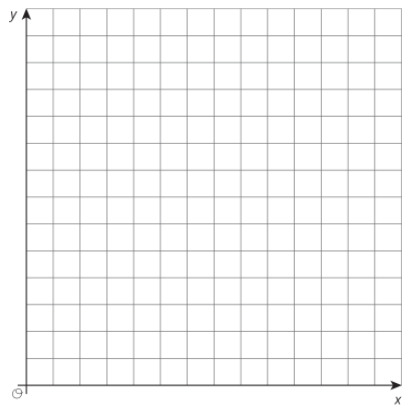Draw your graph on paper, take a picture, and upload it using the image upload icon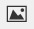.

If you do not have the ability to upload an image of your work type "Graph is on paper."

Part A)

The graph shows the height in inches, h, of a bamboo plant t months after it has been planted.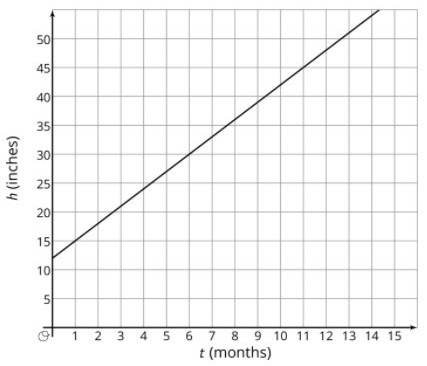Write an equation that describes the relationship between h and t.

Complete the equation below

h = _______________

Part B)

After how many months will the bamboo plant be 66 inches tall?

Type your answer below as a number (example: 5, 3.1, 4 1/2, or 3/2):
Part C)

Part A)

Here are recipes for two different banana cakes. Information for the first recipe is shown in the table.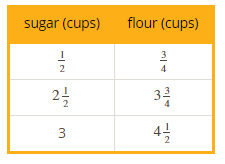The relationship between cups of flour y and cups of sugar x in the second recipe is y=x

If you used 4 cups of sugar, how many cups of flour does the first recipe need?

Type your answer below as a number (example: 5, 3.1, 4 1/2, or 3/2):
Part B)

If you used 4 cups of sugar, how many cups of flour does the second recipe need?

Type your answer below as a number (example: 5, 3.1, 4 1/2, or 3/2):
Part C)

What is the constant of proportionality for the first situation?

Do not include units (cups of flour per cup of sugar) in your answer.

Type your answer below as a number (example: 5, 3.1, 4 1/2, or 3/2):
Part D)

What does it mean?

Part E)

What is the constant of proportionality for the second situation?

Do not include units (cups of flour per cup of sugar) in your answer.

Type your answer below as a number (example: 5, 3.1, 4 1/2, or 3/2):
Part F)

What does it mean?